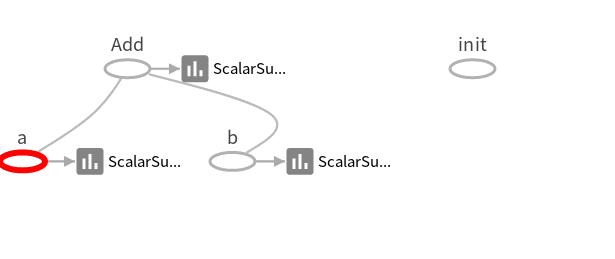• 建立一个计算图
• 初始化变量
• 创建会话
• 在会话中运行图
• 关闭会话

import tensorflow as tf

a=tf.placeholder(tf.int16,name='a')
b=tf.placeholder(tf.int16,name='b')
init = tf.initialize_all_variables()

tf.scalar_summary('a',a)
tf.scalar_summary('b',b)
merged = tf.merge_all_summaries()
with tf.Session() as sess:
writer=tf.train.SummaryWriter('/tmp/tensorflow-start',sess.graph)
#sess.run(init)
result = sess.run(merged,feed_dict={a:2,b:3})